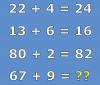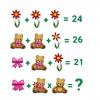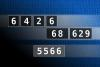BRAIN TEASERSBrain Teasers User Profile

# Sohail Zafar

rank
336
points
8
See full ranking list
short ranking list
 334 Anish Karki 8 335 Sherly Clement 8 336 Sohail Zafar 8 337 Krishna Rao 8 338 Lucky Mishra 8
 Mathematical Puzzle: IF 22+4... Mathematical Puzzle: IF 22+4=24, 13+6=16 and 80+2=82 THEN 67+9=?Can you replace the question mark with a number? MATH PUZZLE: Can you replace the question mark with a number?Calculate the number 5566 NUMBERMANIA: Calculate the number 5566 using numbers [6, 4, 2, 6, 68, 629] and basic arithmetic operations (+, -, *, /). Each of the numbers can be used only once.